Wiki Home Members page Loan function

### Loan function

2023-03-30
Loan

iCafeCloud provides a loan function which means the owner can let members play on credit. To use loan function, follow the steps below:

1. Go to the members page and right click on a member and click on “Add Loan” (Figure 1).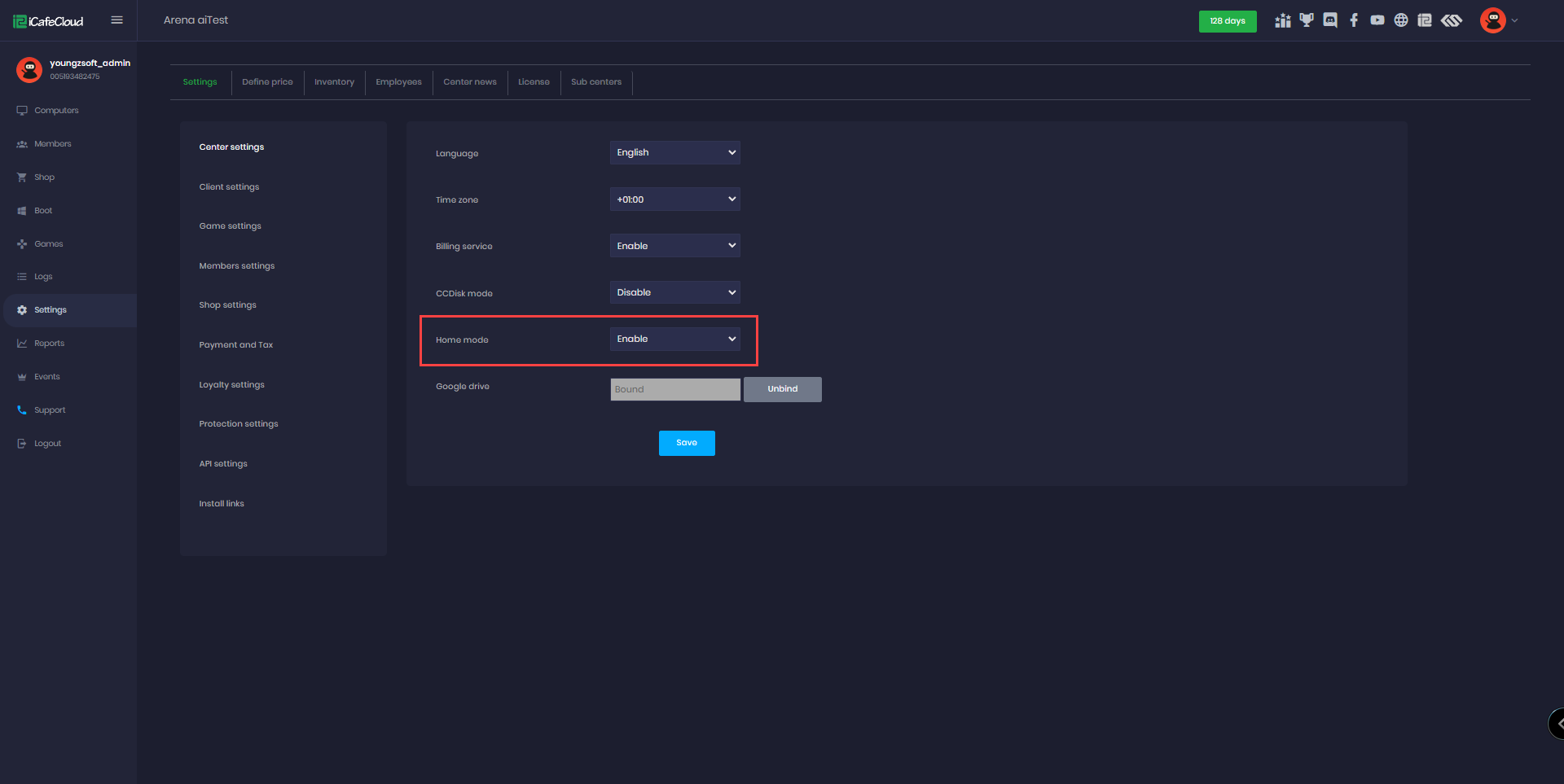Figure 1

1. Enter the loan amount and click on the “Submit” button (Figure 2).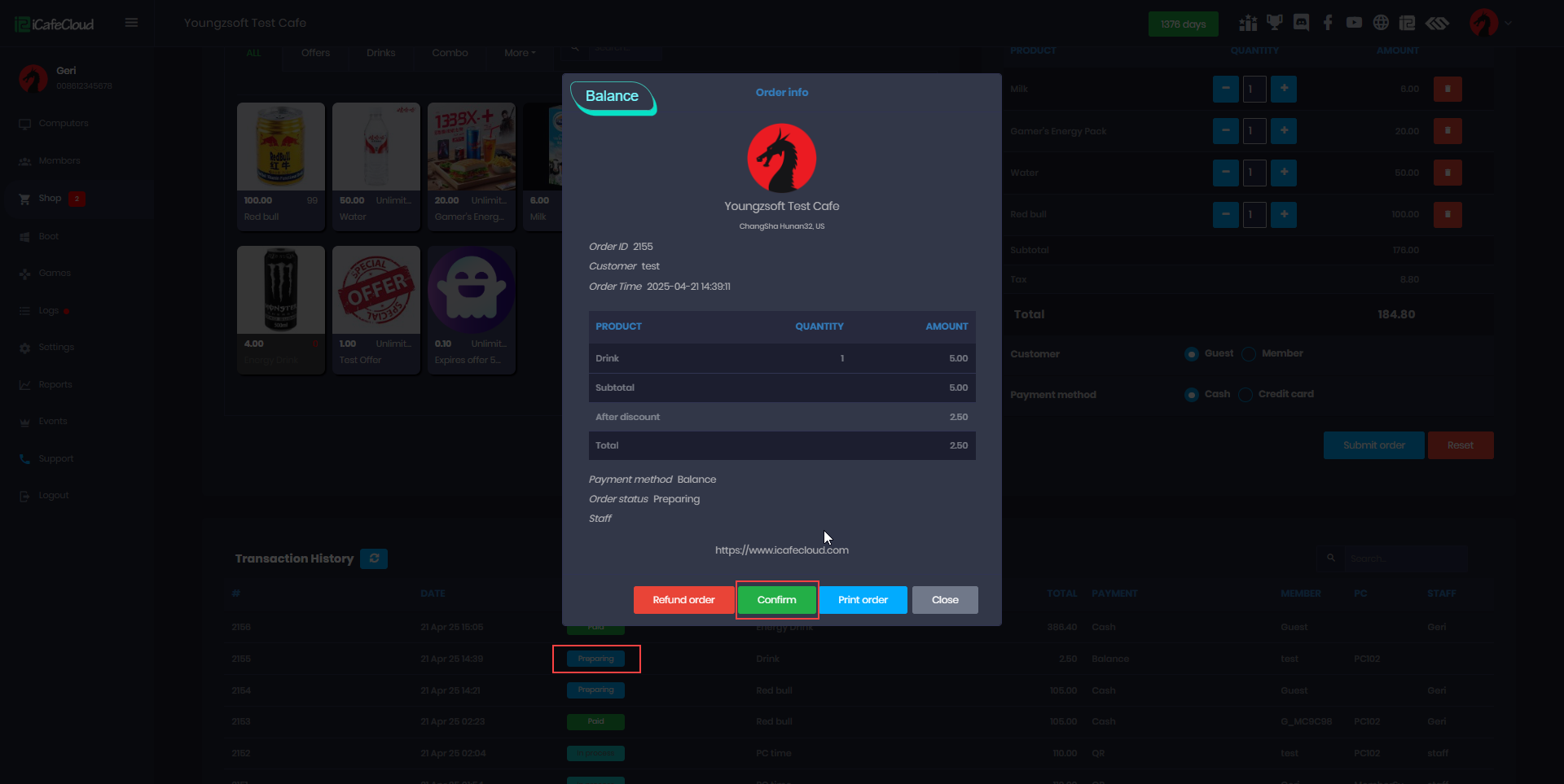Figure 2

1. The loan amount will be shown in the Loan column on the members page and amount will be added to members balance (Figure 3).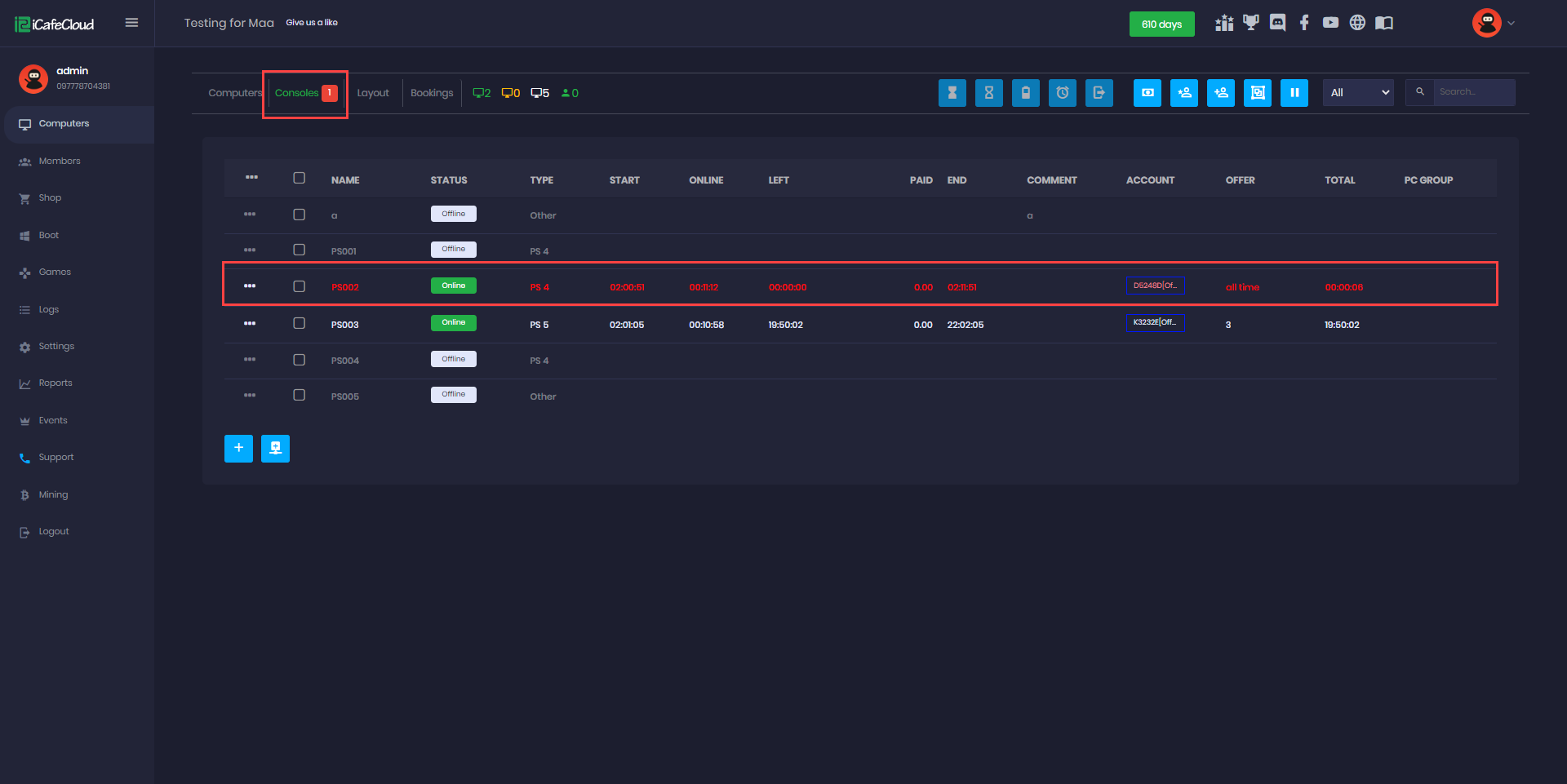Figure 3

Loan amount is deducted from the total balance when the member topup his account. The loan amount is deducted first and if any excess then, it will be added to the balance.

Here is an example:

The member has a loan of 600 and current balance of 450 (Figure 4)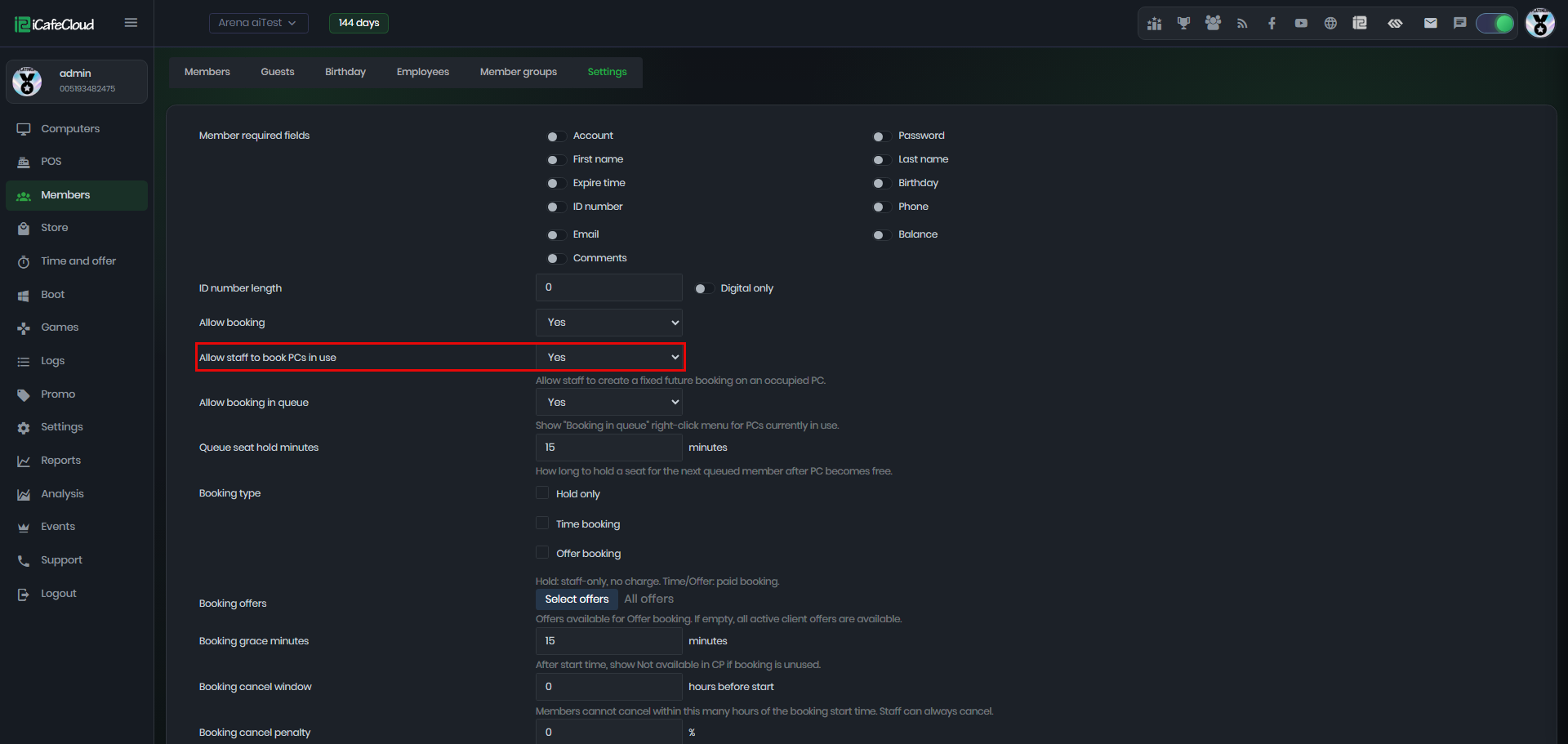Figure 4

Now the member tops up his account 100 (Figure 5).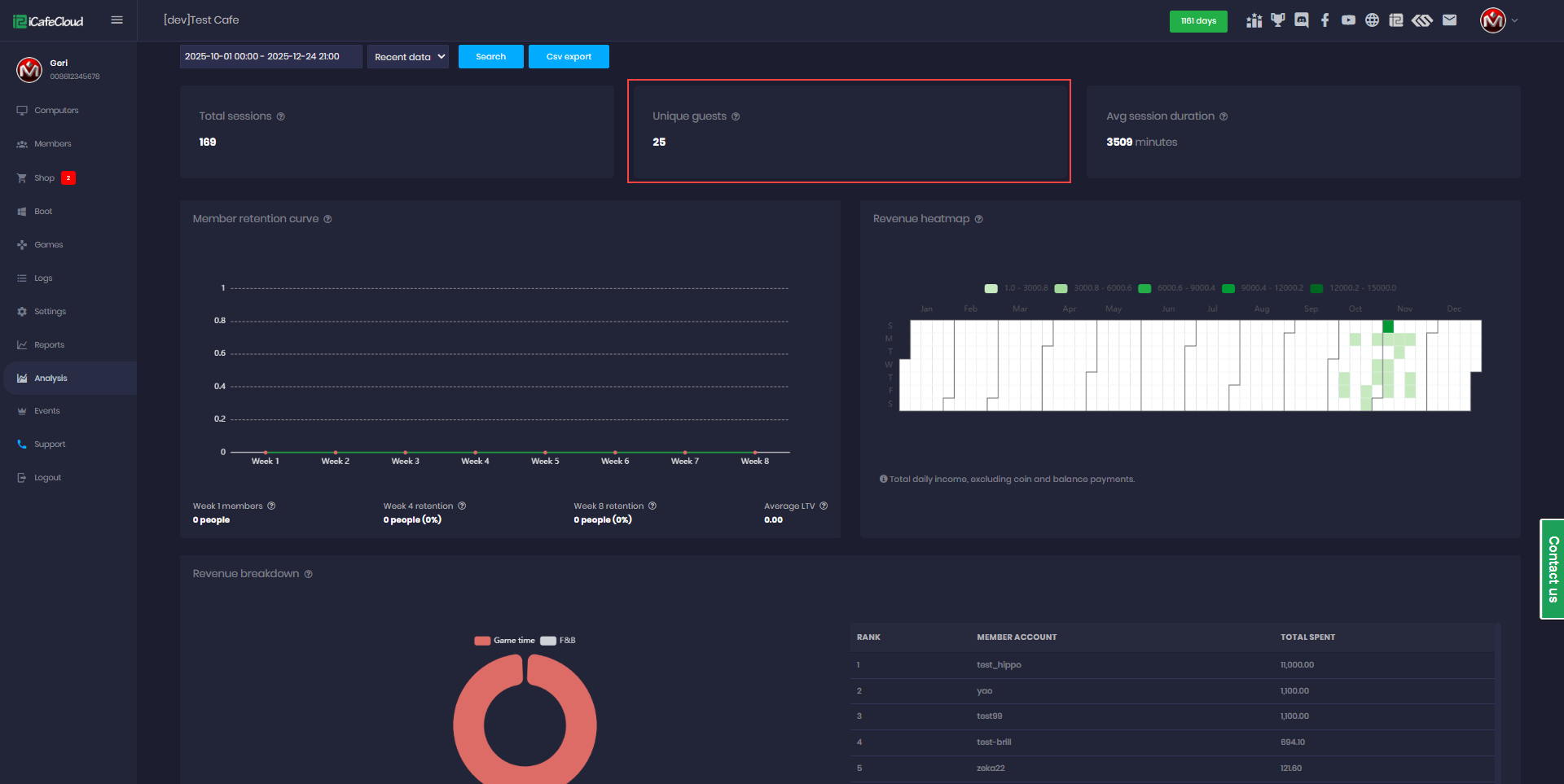Figure 5

After top up, his new balance is 0, because the previous balance of 450 plus 100 (last top up) is deducted from the loan amount and remaining loan balance is 50 (Figure 6).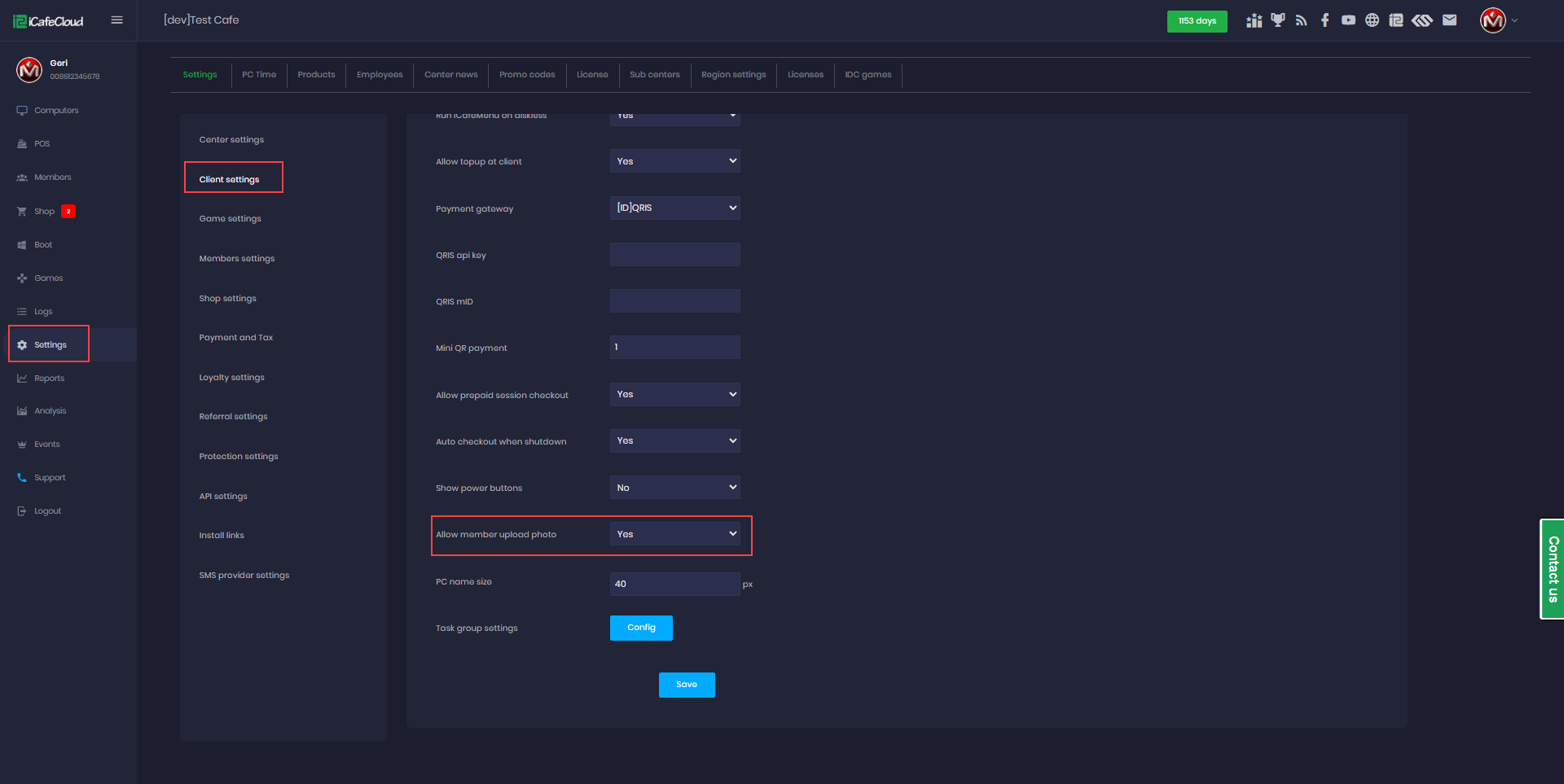Figure 6

Let see again, the member tops up 100 balance again (Figure 7).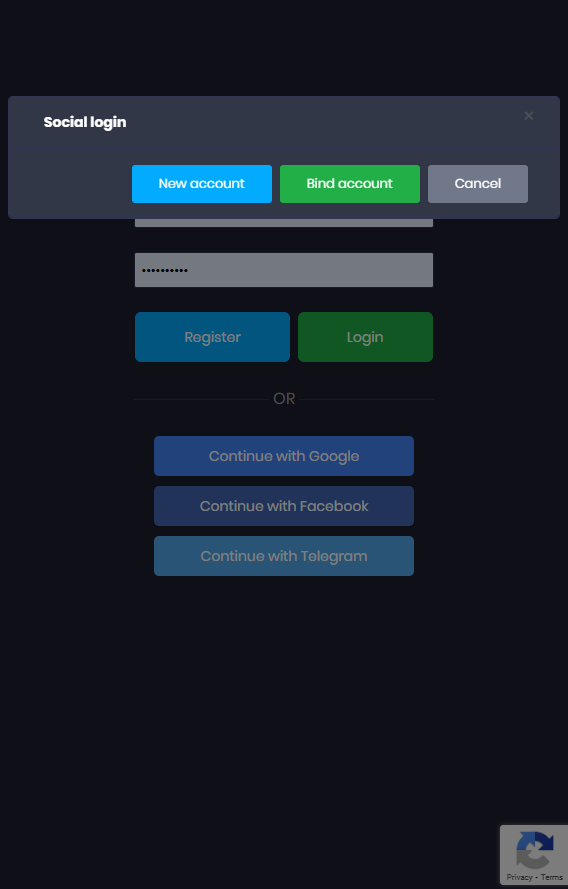Figure 7

The member's balance will be 100, but he has a loan of 50, which is deducted from the balance and so the final balance becomes 50 and loan is 0 (Figure 8).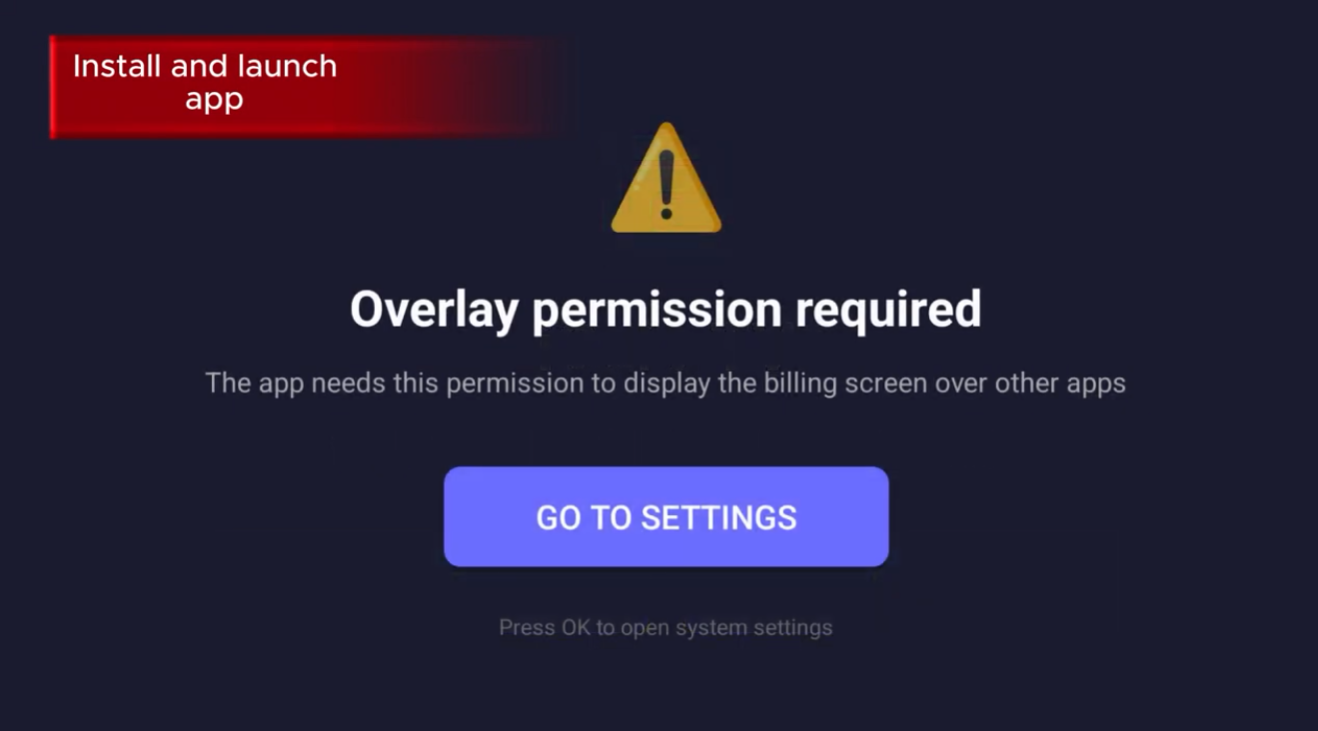Figure 8